# Introduction to Wireless Sensor Networks Routing in WSNs

• Slides: 23
Download presentation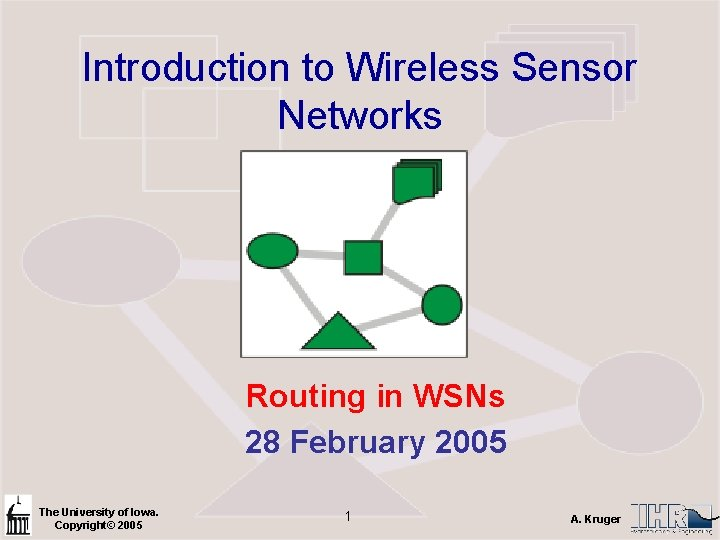Introduction to Wireless Sensor Networks Routing in WSNs 28 February 2005 The University of Iowa. Copyright© 2005 1 A. KrugerOrganizational Class Website www. engineering. uiowa. edu/~ece 195/2005/ Class Time Monday 4: 30 -5: 20 Room 4511 SC Thursday 12: 30 -1: 20 Room 3220 SC Please note that the room numbers are different for Mondays and Thursdays. Office Hours Monday 5: 20 -620 Room 1126 SC Thursday 1: 30 -2: 30 Room 1126 SC Midterm Exam Time: March 10, 2005 The University of Iowa. Copyright© 2005 2 A. KrugerRouting • What is meant by “routing”? • Internet (TCP/IP) – Routing tables often large – Can be updated frequently • WSN – Frequent topology changes – Modest local storage – Expensive to update frequently – => Need local, stateless algorithms where nodes know only immediate neighbors The University of Iowa. Copyright© 2005 3 A. Kruger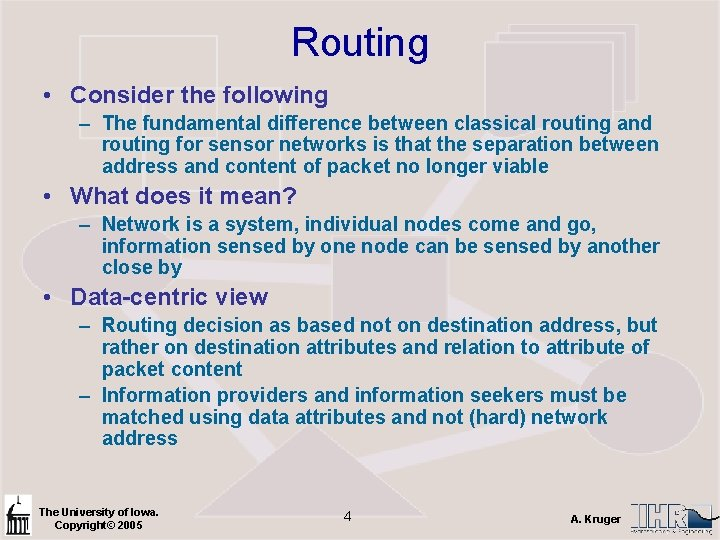Routing • Consider the following – The fundamental difference between classical routing and routing for sensor networks is that the separation between address and content of packet no longer viable • What does it mean? – Network is a system, individual nodes come and go, information sensed by one node can be sensed by another close by • Data-centric view – Routing decision as based not on destination address, but rather on destination attributes and relation to attribute of packet content – Information providers and information seekers must be matched using data attributes and not (hard) network address The University of Iowa. Copyright© 2005 4 A. Kruger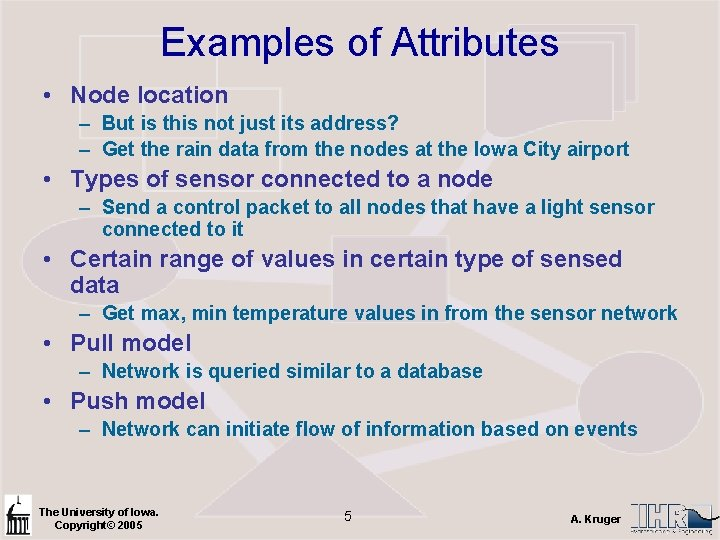Examples of Attributes • Node location – But is this not just its address? – Get the rain data from the nodes at the Iowa City airport • Types of sensor connected to a node – Send a control packet to all nodes that have a light sensor connected to it • Certain range of values in certain type of sensed data – Get max, min temperature values in from the sensor network • Pull model – Network is queried similar to a database • Push model – Network can initiate flow of information based on events The University of Iowa. Copyright© 2005 5 A. Kruger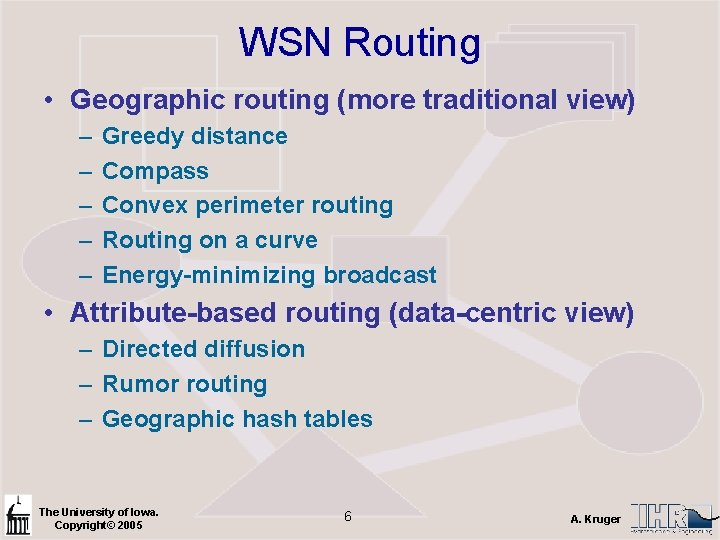WSN Routing • Geographic routing (more traditional view) – – – Greedy distance Compass Convex perimeter routing Routing on a curve Energy-minimizing broadcast • Attribute-based routing (data-centric view) – Directed diffusion – Rumor routing – Geographic hash tables The University of Iowa. Copyright© 2005 6 A. Kruger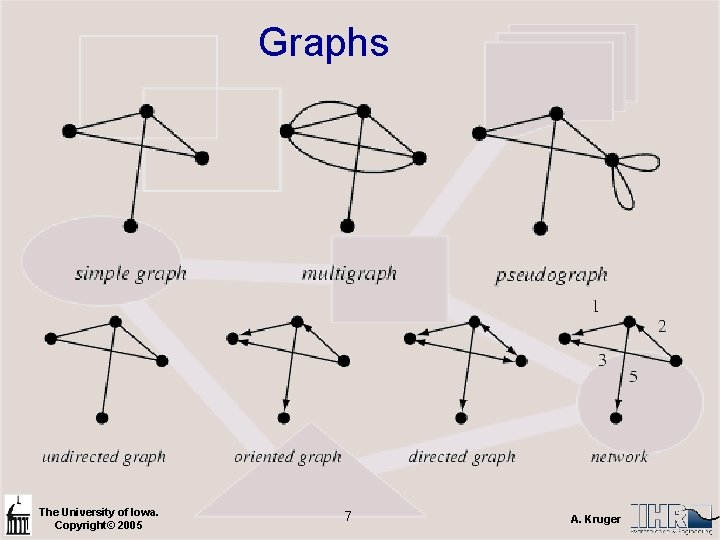Graphs The University of Iowa. Copyright© 2005 7 A. KrugerGreedy Distance and Compass Routing • Greedy distance –pick the locally optimum (distance) neighbor • Compass routing – pick the locally optimum (angle) neighbor The University of Iowa. Copyright© 2005 8 A. KrugerProblem With Greedy Distance • Here both x’s neighbors are further than destination The University of Iowa. Copyright© 2005 9 A. Kruger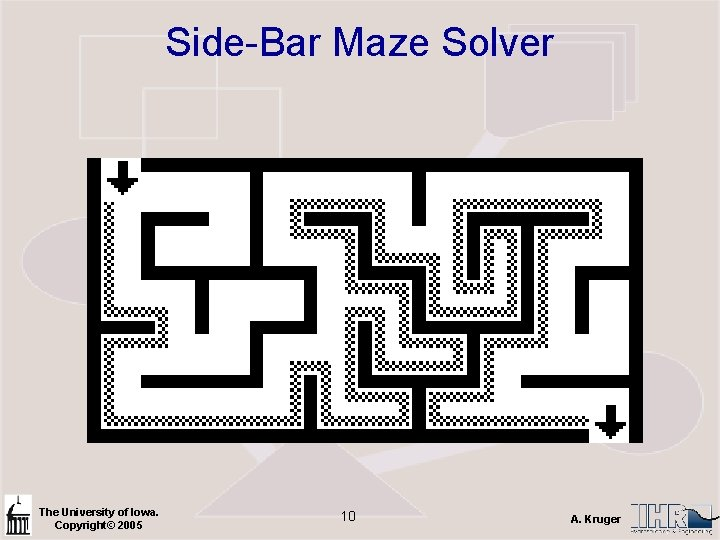Side-Bar Maze Solver The University of Iowa. Copyright© 2005 10 A. Kruger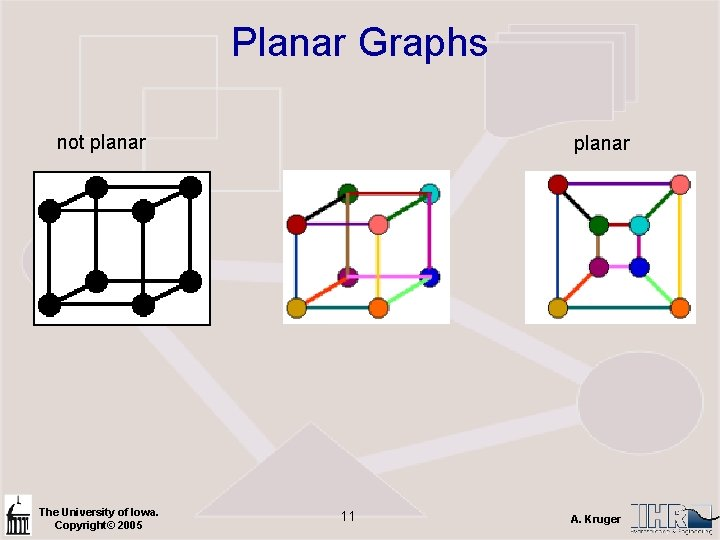Planar Graphs not planar The University of Iowa. Copyright© 2005 planar 11 A. KrugerPlanerization Basic idea – keep connectivity between nodes Convex Polygon Concave Polygon The University of Iowa. Copyright© 2005 12 A. Kruger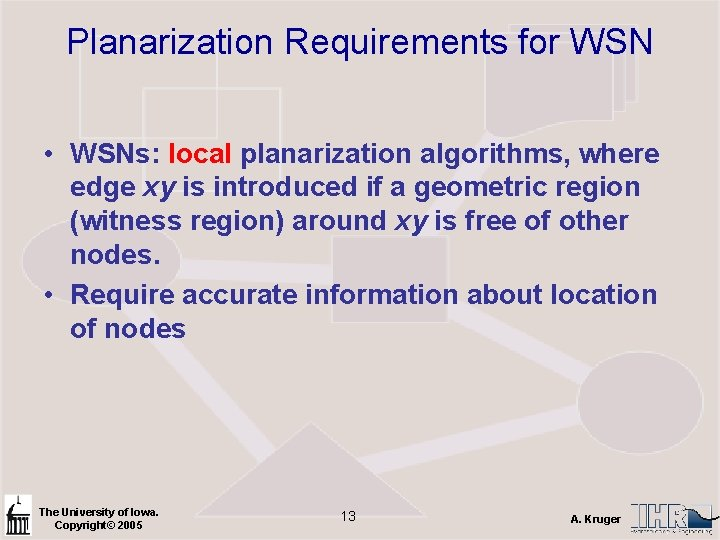Planarization Requirements for WSN • WSNs: local planarization algorithms, where edge xy is introduced if a geometric region (witness region) around xy is free of other nodes. • Require accurate information about location of nodes The University of Iowa. Copyright© 2005 13 A. Kruger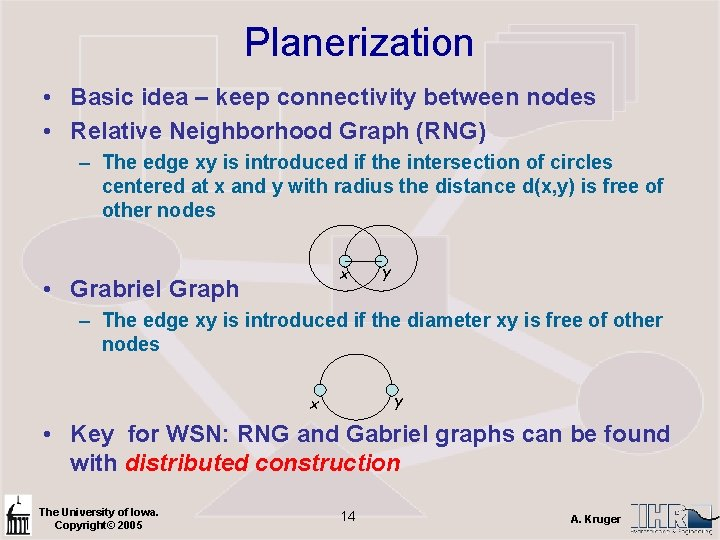Planerization • Basic idea – keep connectivity between nodes • Relative Neighborhood Graph (RNG) – The edge xy is introduced if the intersection of circles centered at x and y with radius the distance d(x, y) is free of other nodes x • Grabriel Graph y – The edge xy is introduced if the diameter xy is free of other nodes y x • Key for WSN: RNG and Gabriel graphs can be found with distributed construction The University of Iowa. Copyright© 2005 14 A. KrugerExamples RNG The University of Iowa. Copyright© 2005 Gabriel 15 A. Kruger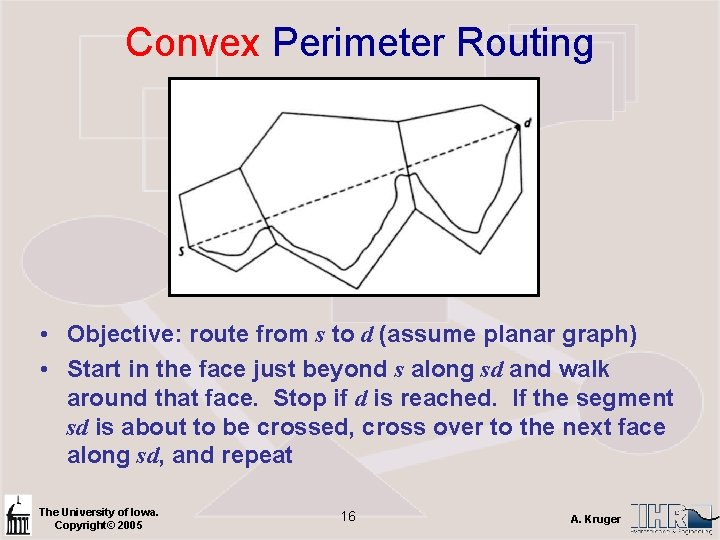Convex Perimeter Routing • Objective: route from s to d (assume planar graph) • Start in the face just beyond s along sd and walk around that face. Stop if d is reached. If the segment sd is about to be crossed, cross over to the next face along sd, and repeat The University of Iowa. Copyright© 2005 16 A. KrugerVariations • Non-convex routing adaptation • OFR – Other face routing The University of Iowa. Copyright© 2005 17 A. KrugerSide-Bar Parametric Equations • Circle – Non parametric: x 2 + y 2 = a 2 – Parametric: x = a cos(t), y = a sin(t), t the parameter • Straight Line – Non parametric: y = mx+c – Parametric: line through point (a, b) parallel to vector (u, v) is given by (x, y) = (a, b) + t·(u, v), t the parameter • Given t one can compute x and y The University of Iowa. Copyright© 2005 18 A. Kruger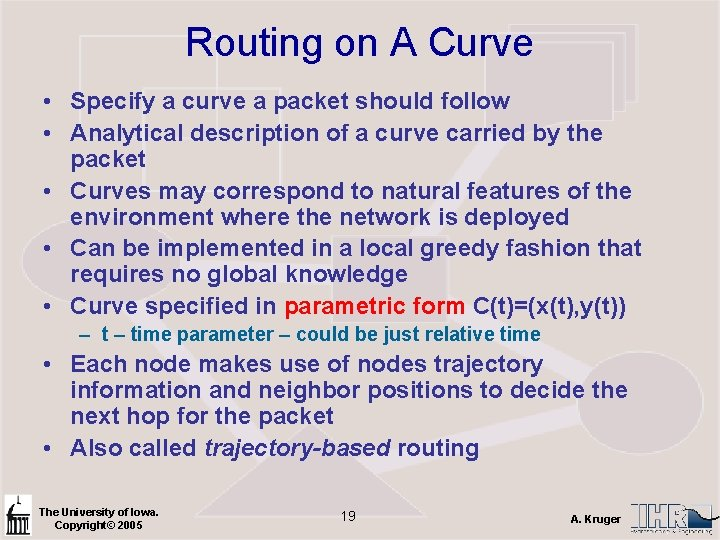Routing on A Curve • Specify a curve a packet should follow • Analytical description of a curve carried by the packet • Curves may correspond to natural features of the environment where the network is deployed • Can be implemented in a local greedy fashion that requires no global knowledge • Curve specified in parametric form C(t)=(x(t), y(t)) – time parameter – could be just relative time • Each node makes use of nodes trajectory information and neighbor positions to decide the next hop for the packet • Also called trajectory-based routing The University of Iowa. Copyright© 2005 19 A. KrugerThe University of Iowa. Copyright© 2005 20 A. KrugerOptimal Path • What do we mean by “optimal” – Minimum delay => fewest hops – Minimum Energy => frequent hops (why) • Formally, cost of a path – – – Where l(e) is the length of the edge in the graph k is in range 1… 5 k = 0 => Hop length, measure delay k = 1 => Euclidian path length k > 1 => Capture energy of path, depending on attenuation model The University of Iowa. Copyright© 2005 21 A. Kruger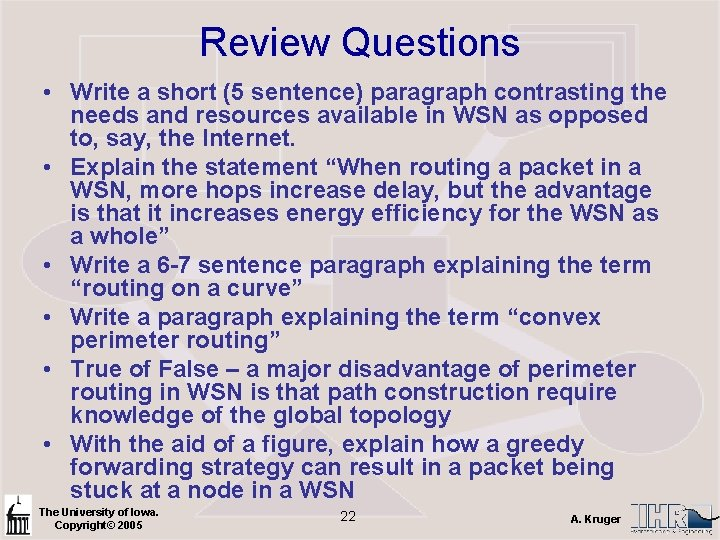Review Questions • Write a short (5 sentence) paragraph contrasting the needs and resources available in WSN as opposed to, say, the Internet. • Explain the statement “When routing a packet in a WSN, more hops increase delay, but the advantage is that it increases energy efficiency for the WSN as a whole” • Write a 6 -7 sentence paragraph explaining the term “routing on a curve” • Write a paragraph explaining the term “convex perimeter routing” • True of False – a major disadvantage of perimeter routing in WSN is that path construction require knowledge of the global topology • With the aid of a figure, explain how a greedy forwarding strategy can result in a packet being stuck at a node in a WSN The University of Iowa. Copyright© 2005 22 A. KrugerReview Questions • Below is a connectivity graph for a WSN. (a) Planerize it using the RNG method. Planerize it using the Grabriel method. (figure goes here) • True or False – a problem with “Routing on a Curve” is that each nodes must know the location of all nodes along the routing path. • Write a short (5 sentence) paragraph explaining what Trajectory-Based Routing is. The University of Iowa. Copyright© 2005 23 A. Kruger